Question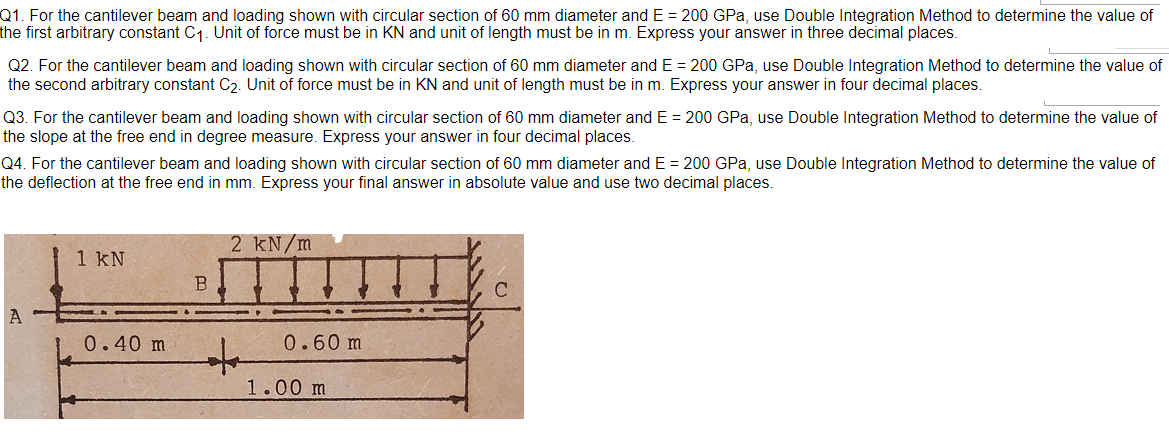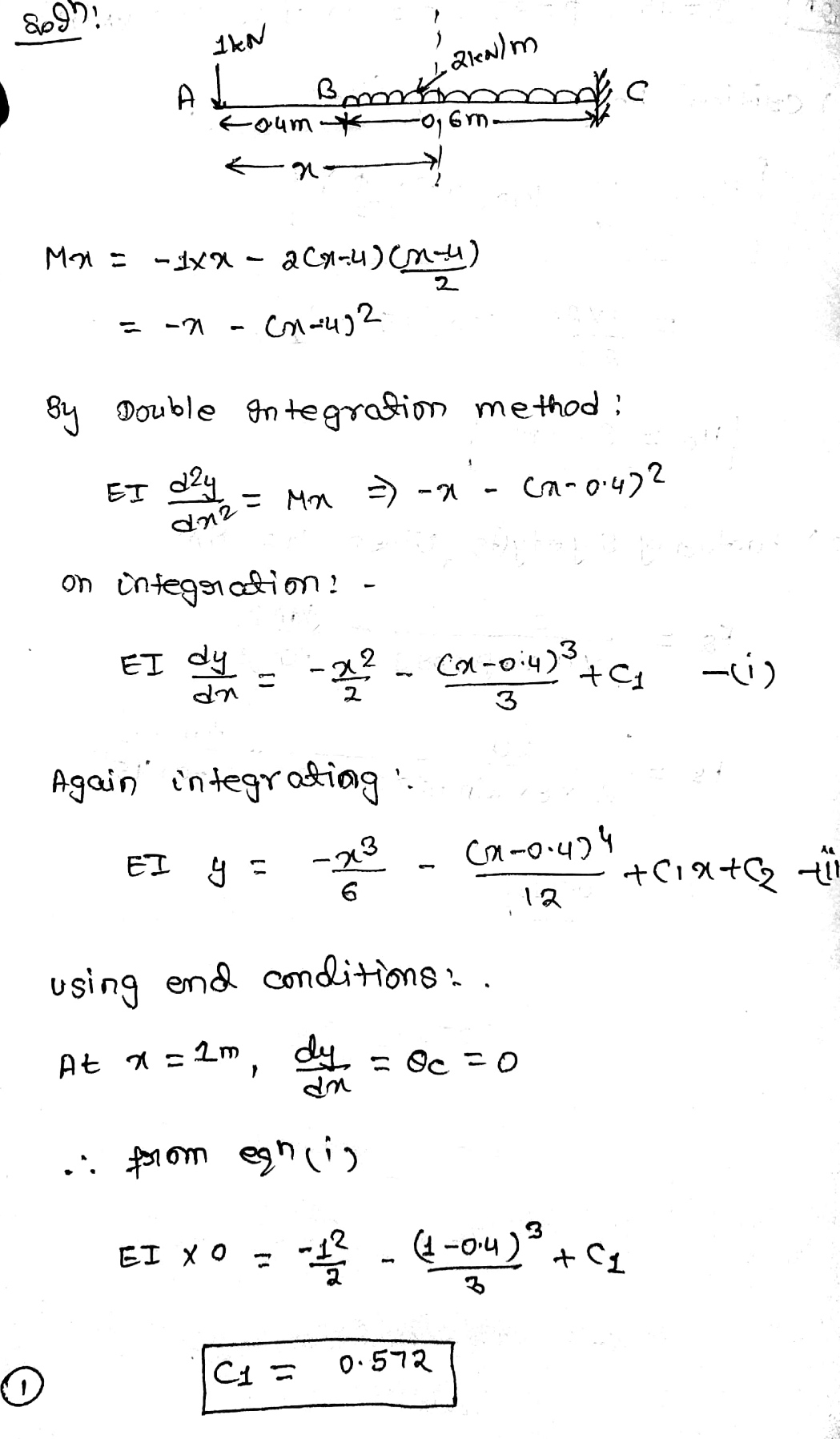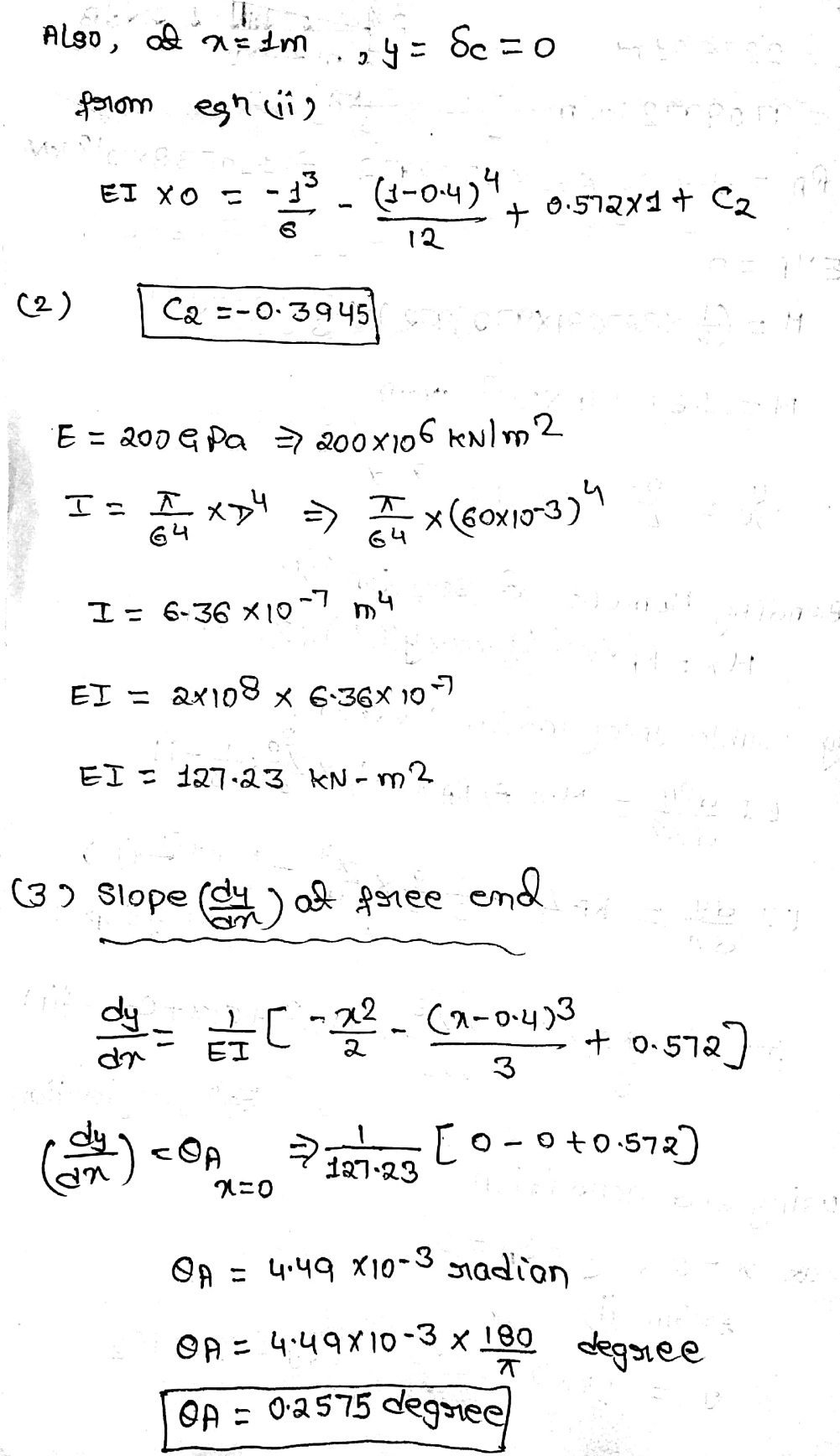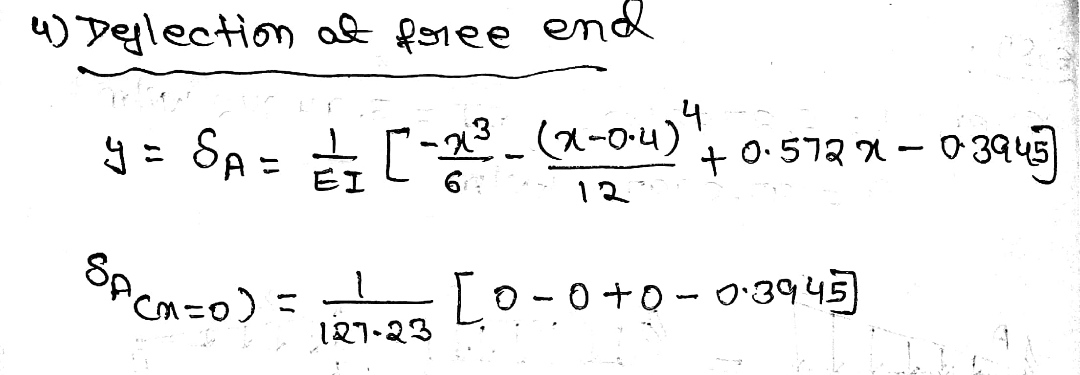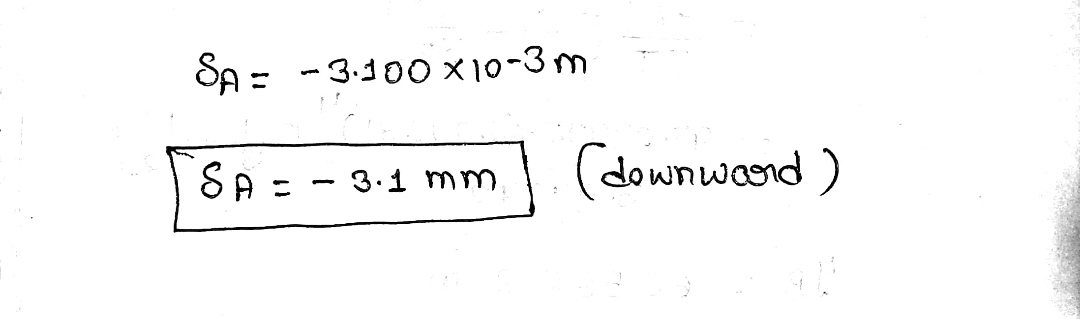#### Earn Coins

Coins can be redeemed for fabulous gifts.

Similar Homework Help Questions
• ### For the cantilever beam and loading shown, determine the slope and deflection at point B. Use...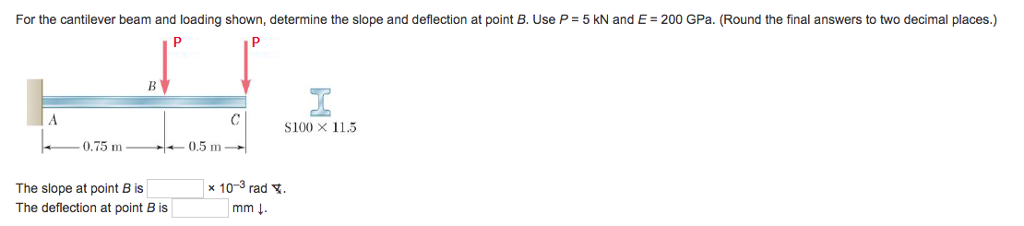For the cantilever beam and loading shown, determine the slope and deflection at point B. Use P 5 kN and E 200 GPa. (Round the final answers to two decimal places.) S100 X 11.5 0.75 m 0.5 m The slope at point B is The deflection at point B is x 10-3 rad. mm ↓

• ### For the cantilever beam and loading shown, use the method of superposition to determine (a) the...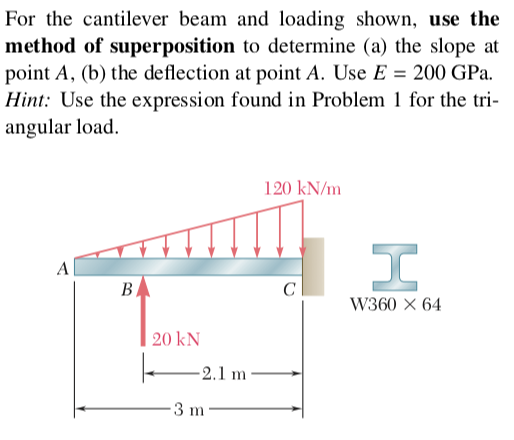For the cantilever beam and loading shown, use the method of superposition to determine (a) the slope at point A, (b) the deflection at point A. Use E 200 GPa. Hint: Use the expression found in Problem 1 for the tri angular load. 120 kN/m W360 × 64 20 kN 2.1 m

• ### Check my work For the cantilever beam and loading shown, determine the slope and deflection at...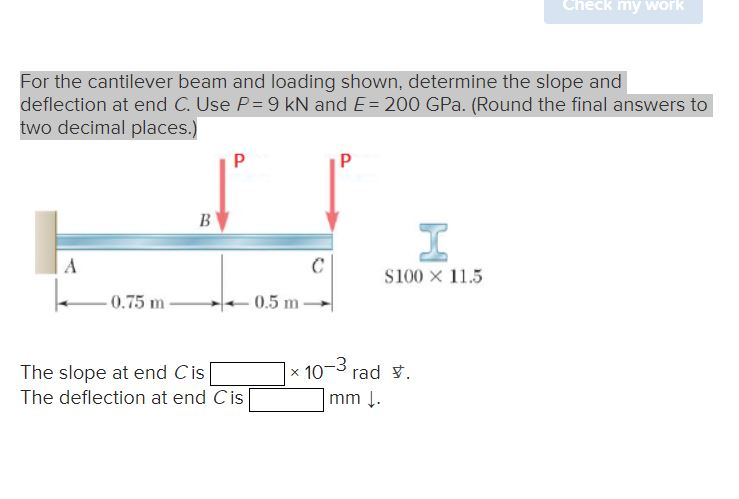Check my work For the cantilever beam and loading shown, determine the slope and deflection at end C. Use P = 9 kN and E= 200 GPa. (Round the final answers to two decimal places.) P Р B I A \$100 X 11.5 -0.75 m 0.5 m The slope at end Cis The deflection at end Cis x 10m rad . -3 mm.

• ### Question 4 (25 marks) For the beam and loading shown in Figure 4, knowing that a...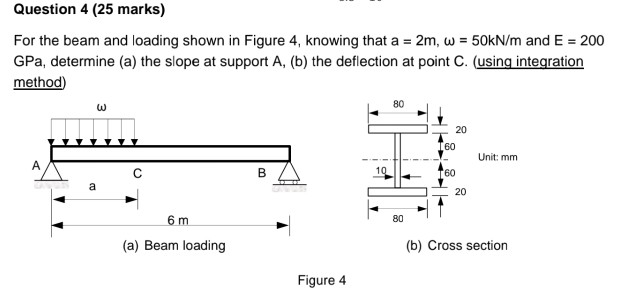Question 4 (25 marks) For the beam and loading shown in Figure 4, knowing that a GPa, determine (a) the slope at support A, (b) the deflection at point C. (using integration method) 2m, w 50KN/m and E 200 80 w 20 60 Unit: mm A 10 60 В а 20 6 m 80 (a) Beam loading (b) Cross section Figure 4

• ### For the cantilever steel beam [E 190 GPa; deflection VA at A 110 x 106 mm*],...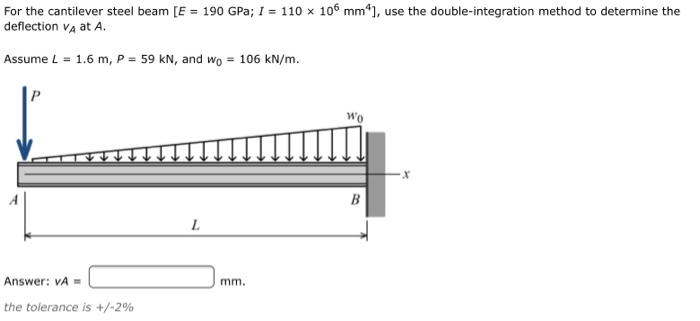For the cantilever steel beam [E 190 GPa; deflection VA at A 110 x 106 mm*], use the double-integration method to determine the Assume L = 1.6 m, P = 59 kN, and wo = 106 kN/m. Answer: VA = the tolerance is +/-296

• ### 2. For the cantilever beam and loading 165 GPa. Shown, calculate the maximum deflection of the...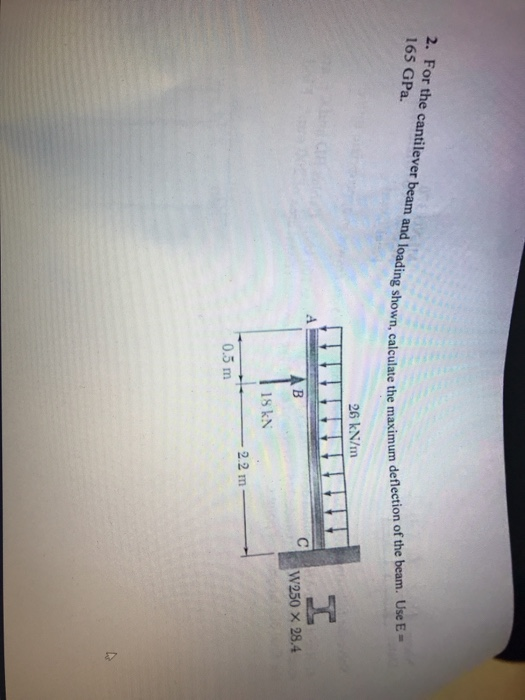2. For the cantilever beam and loading 165 GPa. Shown, calculate the maximum deflection of the beam. Use E- cal 26 kN/m W250 x 28.4 s kN 2.2 m 0.5 m

• ### The cross section of the cantilever beam loaded as shown in Fig. 8-20 is rectangular, 50 × 75 mm. The bar, 1 m long, i...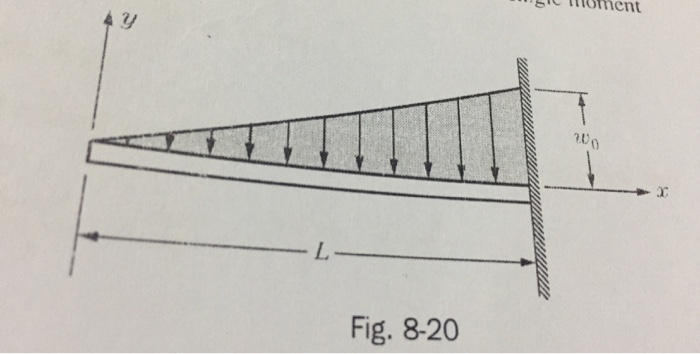The cross section of the cantilever beam loaded as shown in Fig. 8-20 is rectangular, 50 × 75 mm. The bar, 1 m long, is aluminum for which E = 65 GPa. Determine the permissible maximum intensity of loading if the maximum deflection is not to exceed 5 mm and the maximum stress is not to exceed 50 MPa. Ans. w0 = 14.1 kN/m and 17.1 kN/m. Select 14.1 kN/m. oment 3 Fig. 8-20 oment 3 Fig. 8-20

• ### 8. The cantilever beam in Figure Q8 subjects to concentrated loading. The cross section geometry gives...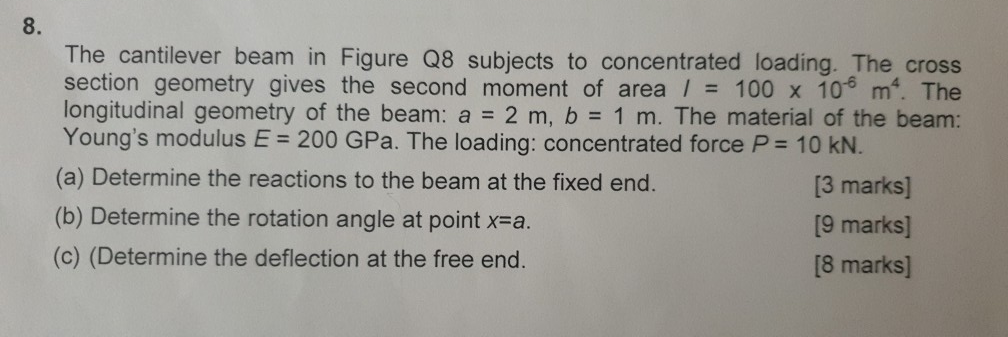8. The cantilever beam in Figure Q8 subjects to concentrated loading. The cross section geometry gives the second moment of area / 100 x 10 m. The longitudinal geometry of the beam: a 2 m, b 1 m. The material of the beam: Young's modulus E 200 GPa. The loading: concentrated force P 10 KN. (a) Determine the reactions to the beam at the fixed end. (b) Determine the rotation angle at point x-a (c) (Determine the deflection at the...

• ### Consider the beam and loading shown. Given: P 8.8 kN 03 m 40 mm 12 mm...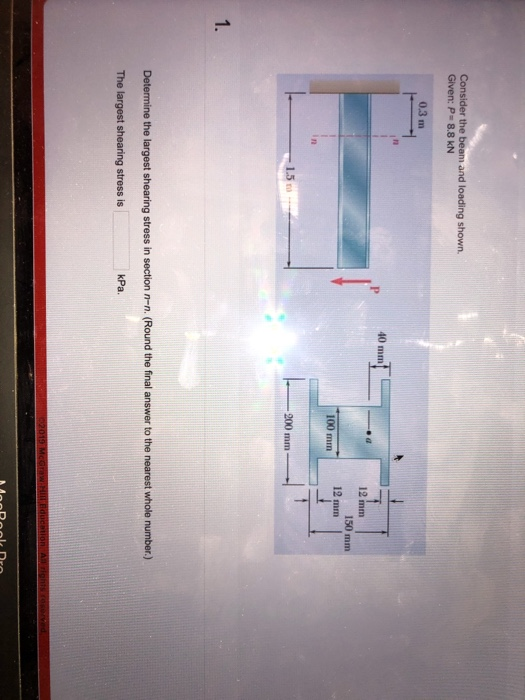Consider the beam and loading shown. Given: P 8.8 kN 03 m 40 mm 12 mm 150 mm 12 mm 100 mm -200 mm 1.5 m 1. Determine the largest shearing stress in section n-n. (Round the final answer to the nearest whole number.) kPa. The largest shearing stress is 02019 McGraw HIll Bducation Al rights cosorved For the beam and loading shown, consider section-nand take P 177 kN. 160 mm 20 mm 100 mm 30 mm 500 mm- 500...

• ### A cantilever beam of a channel section is loaded at its half-length, as shown in Figure Q2. The Young's modulus of the material is 200 GPa. Determine the deflection at the free end. [12.5 marks]...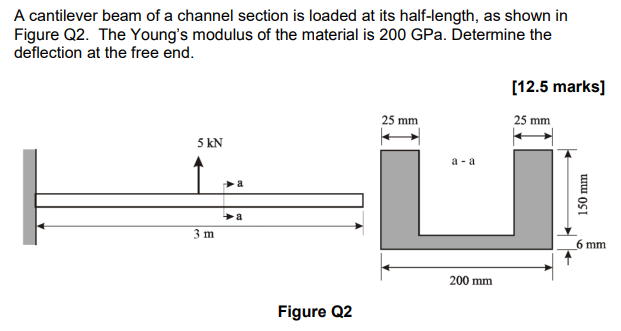A cantilever beam of a channel section is loaded at its half-length, as shown in Figure Q2. The Young's modulus of the material is 200 GPa. Determine the deflection at the free end. [12.5 marks] 25 mm 25 mm 5 kN a -a 少a 6 mm 200 mm Figure Q2 A cantilever beam of a channel section is loaded at its half-length, as shown in Figure Q2. The Young's modulus of the material is 200 GPa. Determine the deflection at...